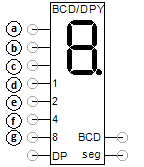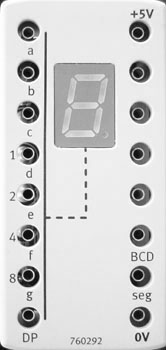# 7-segment displayDecimal and hexadecimal numbers can be displayed with the 7-segment display. Depending on the additional inputs available, the individual segments and a decimal point can be controlled separately. The input DP=1 controls the decimal point.

If the input seg is available and has the value 1, the individual segments are controlled via the inputs a, b, c, d, e, f and g.

If the input seg not available or has the value 0, the number x is displayed, which is composed bit-by-bit from the inputs I1, I2, I4 and I8. If BCD=1 is also set, x is displayed as a decimal number. Otherwise it is displayed as a hexadecimal number. With decimal output, numbers greater than 9 are displayed as -. The following applies for the number x:

x = I1*2^0 + I2*2^1 + I4*2^2 + I8*2^3